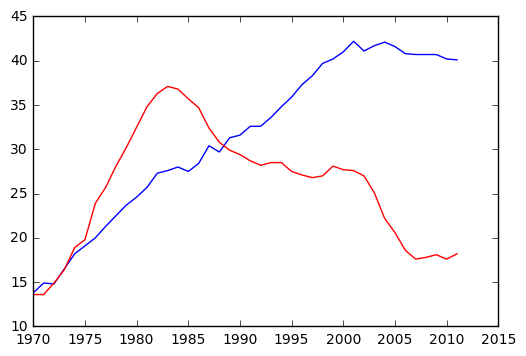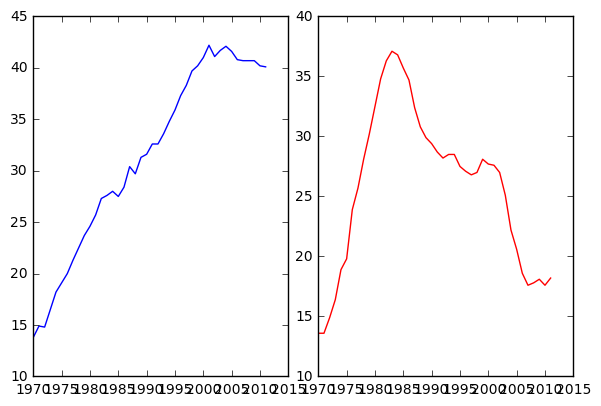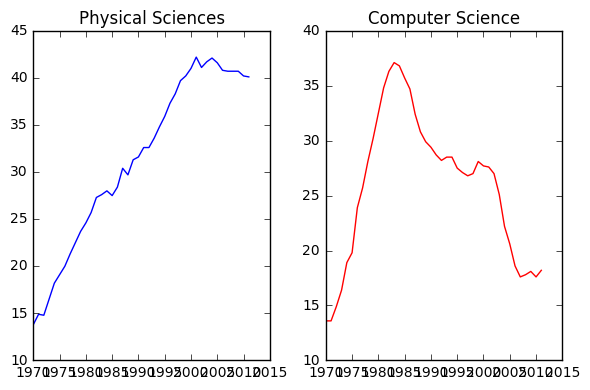# python format print example

In :
print 'before: {:.2f} after'.format(1.5555)

before: 1.56 after

In :
print '{1},{0},{1},{2},{0}'.format('pos',777,True)

777,pos,777,True,pos

In :
print '{name},{age}'.format(age=18,name='cutie')

cutie,18

In :
has=['first', 2.00, 'third']
print '1st {0} all: {0} last {0} end'.format(has)

1st first all: ['first', 2.0, 'third'] last third end

In :
print 'start--- {:,} ---end'.format(9876543210)

start--- 9,876,543,210 ---end

In :
print 'start:{:>8}'.format(123)

start:     123

In :
print 'start:{:0>8}'.format(123)

start:00000123

In :
print 'start:{:A>8}'.format(123)

start:AAAAA123

In [ ]:


In [ ]:


In [ ]:



# understand axis in matplotlib

axis is a subarea within figure() in matplotlib

figure()

plt.axes([0.3, 0.5, 0.4, 0.2])the figure area size is 1, 1 as base

In :
import matplotlib.pyplot as plt
import numpy as np

In :
physical_sciences=[ 13.8,  14.9,  14.8,  16.5,  18.2,  19.1,  20. ,  21.3,  22.5,
23.7,  24.6,  25.7,  27.3,  27.6,  28. ,  27.5,  28.4,  30.4,
29.7,  31.3,  31.6,  32.6,  32.6,  33.6,  34.8,  35.9,  37.3,
38.3,  39.7,  40.2,  41. ,  42.2,  41.1,  41.7,  42.1,  41.6,
40.8,  40.7,  40.7,  40.7,  40.2,  40.1];
computer_science=[ 13.6,  13.6,  14.9,  16.4,  18.9,  19.8,  23.9,  25.7,  28.1,
30.2,  32.5,  34.8,  36.3,  37.1,  36.8,  35.7,  34.7,  32.4,
30.8,  29.9,  29.4,  28.7,  28.2,  28.5,  28.5,  27.5,  27.1,
26.8,  27. ,  28.1,  27.7,  27.6,  27. ,  25.1,  22.2,  20.6,
18.6,  17.6,  17.8,  18.1,  17.6,  18.2]
year=[1970, 1971, 1972, 1973, 1974, 1975, 1976, 1977, 1978, 1979, 1980,
1981, 1982, 1983, 1984, 1985, 1986, 1987, 1988, 1989, 1990, 1991,
1992, 1993, 1994, 1995, 1996, 1997, 1998, 1999, 2000, 2001, 2002,
2003, 2004, 2005, 2006, 2007, 2008, 2009, 2010, 2011]


### two methods to specify many distinct graphs¶

In :
# Plot in blue the % of degrees awarded to women in the Physical Sciences
plt.plot(year, physical_sciences, color='blue')

# Plot in red the % of degrees awarded to women in Computer Science
plt.plot(year, computer_science, color='red')

# Display the plot
plt.show()In :
# Create plot axes for the first line plot
plt.axes([0.05,0.05,0.425,0.9])

# Plot in blue the % of degrees awarded to women in the Physical Sciences
plt.plot(year,physical_sciences, color='blue')

# Create plot axes for the second line plot
plt.axes([.525,0.05,0.425,0.9])

# Plot in red the % of degrees awarded to women in Computer Science
plt.plot(year,computer_science, color='red')

# Display the plot
plt.show()In :
# Create a figure with 1x2 subplot and make the left subplot active
plt.subplot(1,2,1)

# Plot in blue the % of degrees awarded to women in the Physical Sciences
plt.plot(year, physical_sciences, color='blue')
plt.title('Physical Sciences')

# Make the right subplot active in the current 1x2 subplot grid
plt.subplot(1,2,2)

# Plot in red the % of degrees awarded to women in Computer Science
plt.plot(year, computer_science, color='red')
plt.title('Computer Science')

# Use plt.tight_layout() to improve the spacing between subplots
plt.tight_layout()
plt.show()In [ ]:



# Jupyter notebook into post

add that html source code to XYZ Html

embed that to post like

In :
import numpy as np

from bokeh.plotting import figure, show, output_file
from bokeh.io import output_notebook

N = 4000
x = np.random.random(size=N) * 100
y = np.random.random(size=N) * 100
colors = ["#%02x%02x%02x" % (int(r), int(g), 150) for r, g in zip(50+2*x, 30+2*y)]

In :
output_notebook()

p = figure()